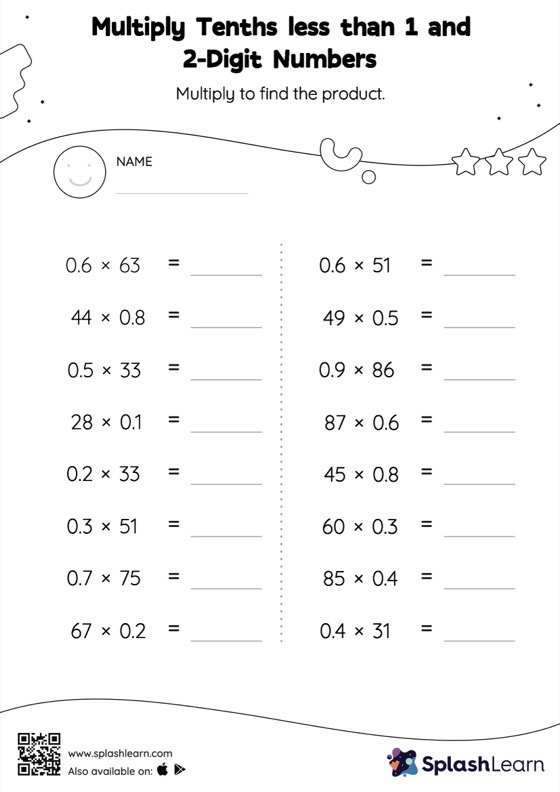# Multiply Tenths less than 1 and 2-Digit Numbers: Horizontal Multiplication Worksheet

Home > Multiply Tenths less than 1 and 2-Digit Numbers: Horizontal MultiplicationHelp your little one develop a knack for math with this multiply tenths less than 1 and 2-digit numbers worksheet. Students may omit the decimal point when multiplying a decimal by a two-digit number and then add it when writing the answer. This multiply tenths less than 1 and 2-digit numbers worksheet gives learners many opportunities to practice this concept. In this worksheet, students practice solving problems written in the horizontal format. How numbers are laid out in a problem affects the method a student employs to solve it. Therefore to develop actual fluency and mastery of multiple strategies, students must practice different formats.